This book contains both practical guides on exploring missing data, as well as some of the deeper details of how `naniar` works to help you better explore your missing data. A large component of this book are the exercises that accompany each section in each chapter.

``library(tidyverse)``
``── Attaching packages ─────────────────────────────────────── tidyverse 1.3.1 ──``
``````✔ ggplot2 3.3.6     ✔ purrr   0.3.4
✔ tibble  3.1.7     ✔ dplyr   1.0.9
✔ tidyr   1.2.0     ✔ stringr 1.4.0
✔ readr   2.1.2     ✔ forcats 0.5.1``````
``````── Conflicts ────────────────────────────────────────── tidyverse_conflicts() ──
``library(naniar)``

Now that we’ve explored some ways to summarise data using nabular data, we are going to explore how you can use `nabular` data to explore how variables vary as other variables go missing. We’ll demonstrate this using ggplot, showing how to visualise densities, boxplots, and some ways of creating multiple plots, for each type of missingness.

## 9.1 Visualizing missings using densities

To begin, we can look at the distribution of temperature using ggplot, placing `Temp` on the X axis, and then using `geom_density()` to visualise temperature as a density, or a distribution.

``````ggplot(airquality,
aes(x = Temp)) +
geom_density()``````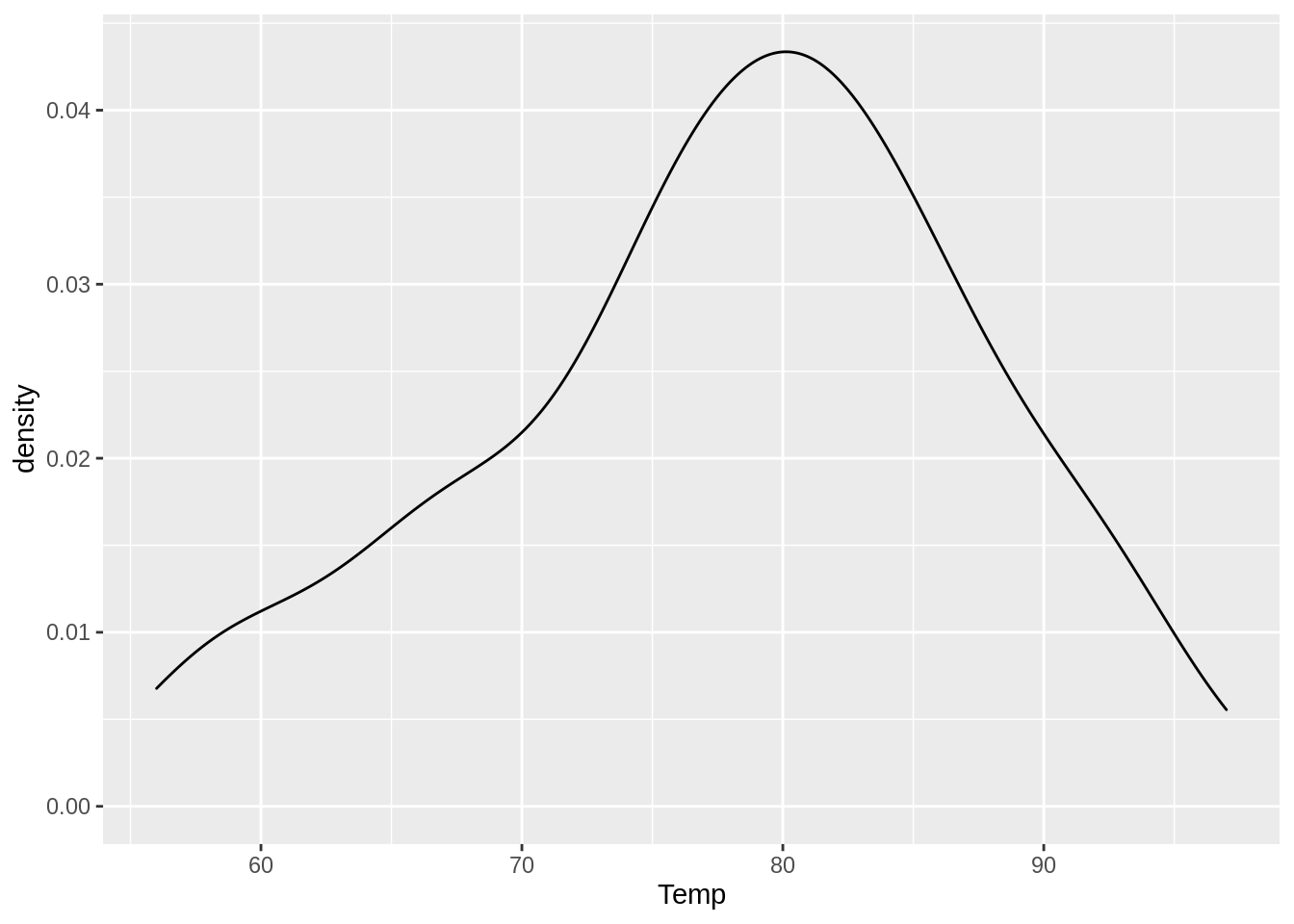To explore how temperature changes when ozone is missing, we create the `nabular` data with `nabular()`, and then add in our aesthetics, `colour = Ozone_NA`.

``````airquality %>%
nabular() %>%
ggplot(aes(x = Temp,
color = Ozone_NA)) +
geom_density()``````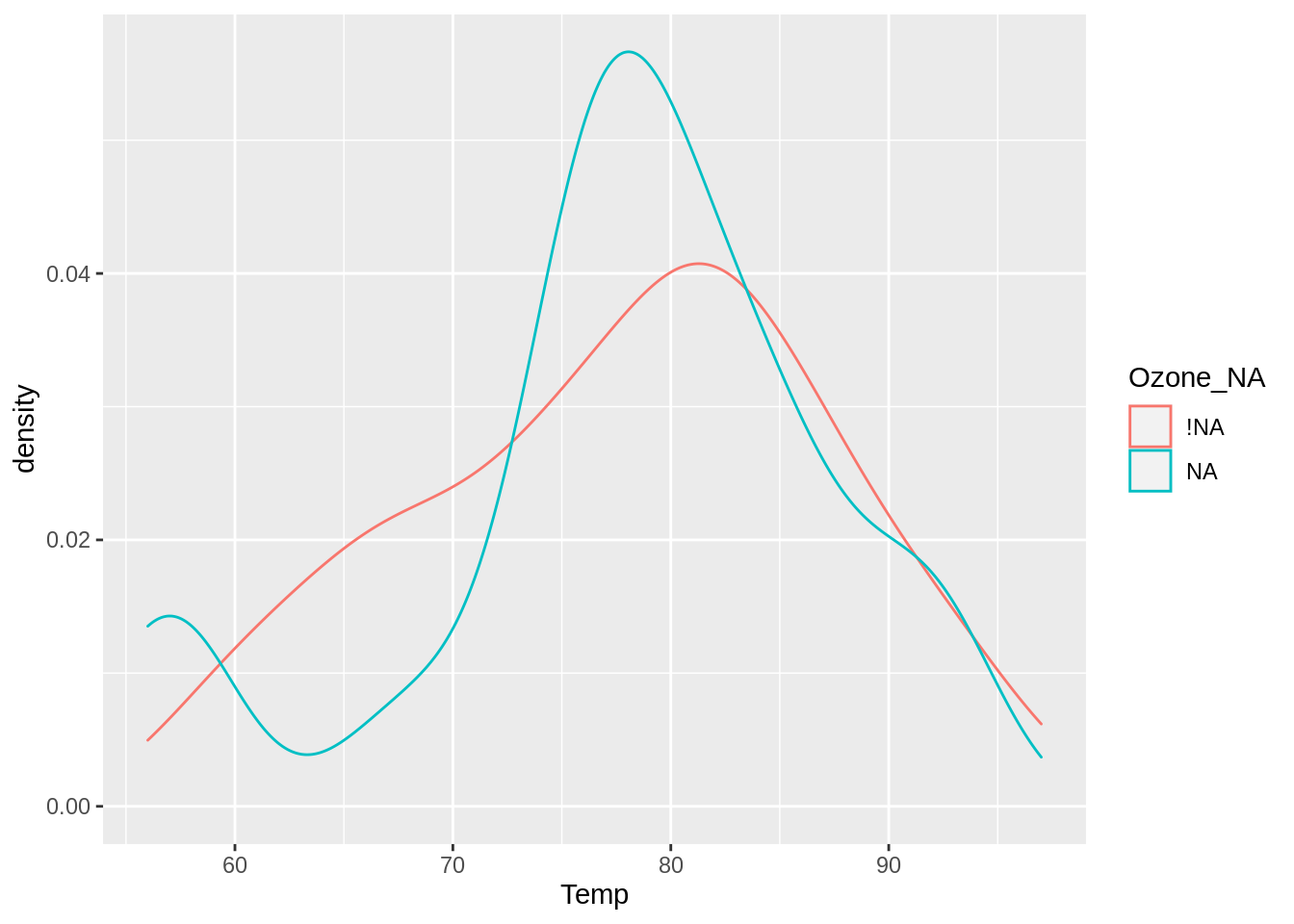This now splits the density into two densities, one for temperature when ozone is present, and one for temperature when ozone is absent. This shows us that the values of temperature don’t change much when ozone is present or absent.

## 9.2 Visualizing missings using boxplots

Similarly, you can use boxplots to explore missing data, by putting the missingness that you would like to explore by on the x axis (`Ozone_NA`), and temperature on the y axis, then using `geom_boxplot()`.

``````airquality %>%
nabular() %>%
ggplot(aes(x = Ozone_NA,
y = Temp)) +
geom_boxplot()``````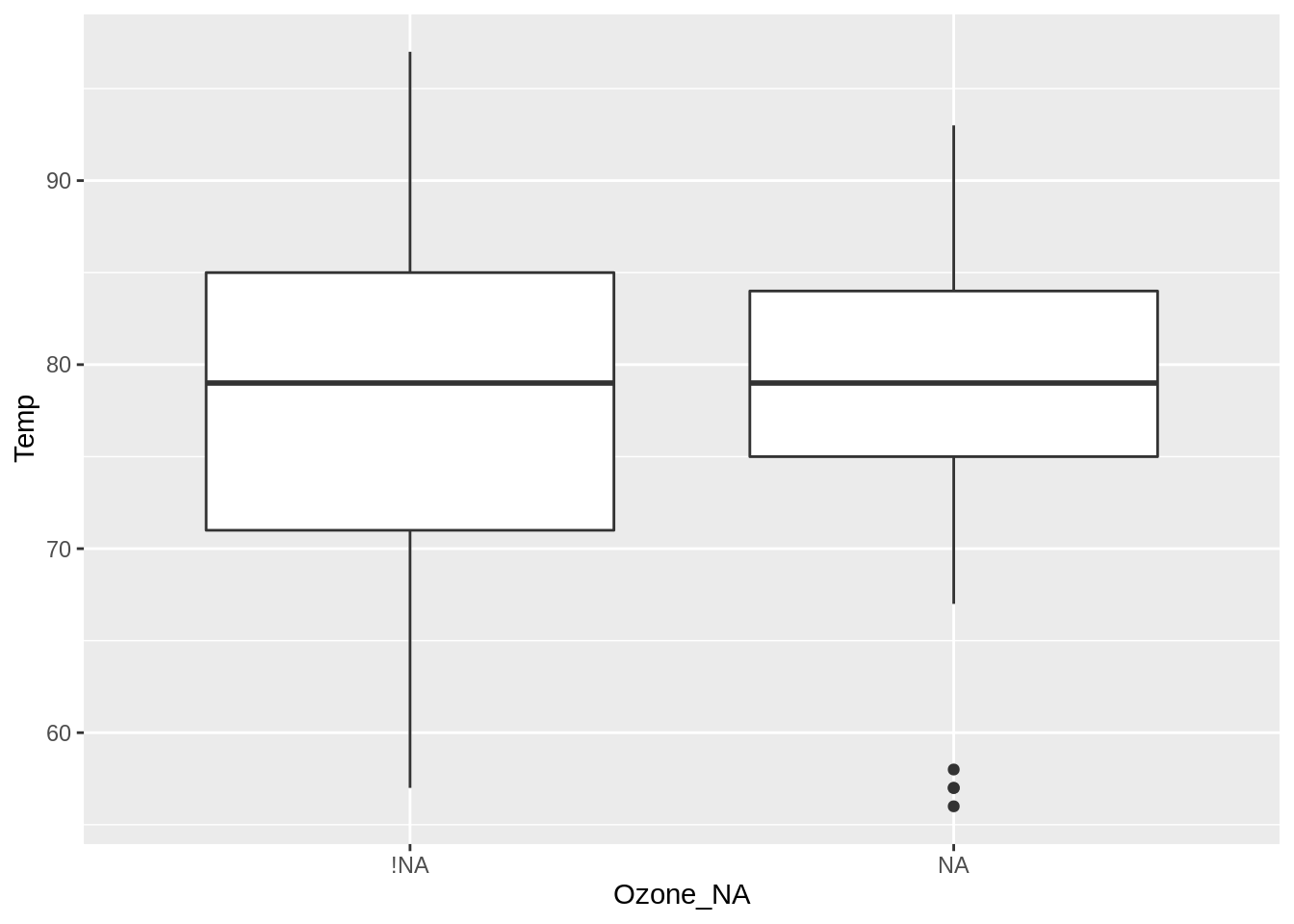What can we learn from this? The values of temperature are similar when ozone is missing versus not missing. However, there is generally less variation for temperature when ozone is missing, but there are also some temperature outliers.

## 9.3 Visualizing missings using facets

We can visualise two densities for temperature according to the missingness of ozone. This is similar to the previous density visualisation, except the densities are not overlaid, and are faceted - they are in separate plots.

A similar visualisation to the previous visualisation of densities can be made using facets. Here, we use `nabular` data to create a density plot, using `facet_wrap(~Ozone_NA)`.

``````airquality %>%
nabular() %>%
ggplot(aes(x = Temp)) +
geom_density() +
facet_wrap(~Ozone_NA)``````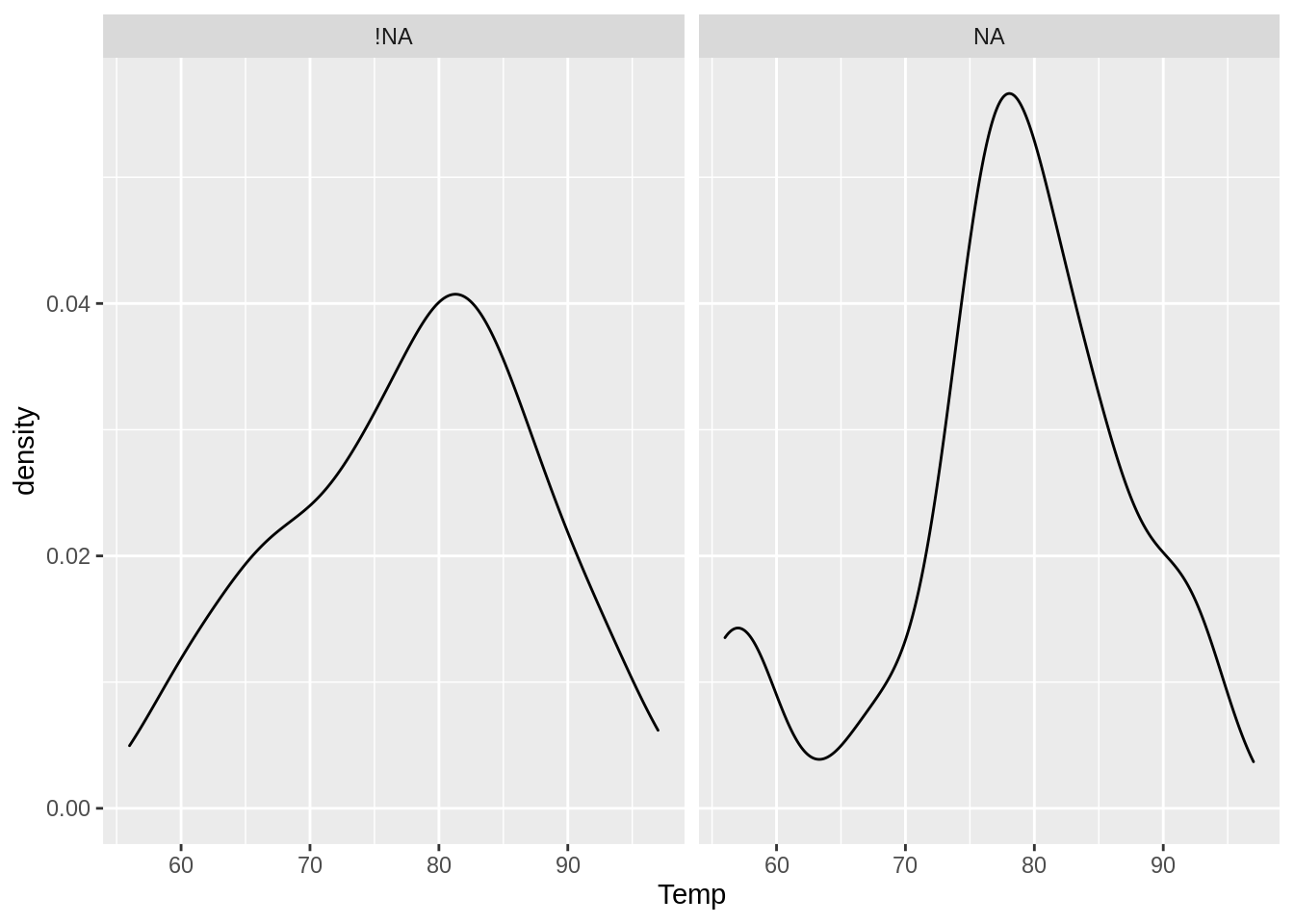Splitting by facet can be useful if you want to compare different types of visualisations.

You can look at two scatterplots, facetting by the missingness of Ozone using `Ozone_NA`, for the values temperature and wind.

``````airquality %>%
nabular() %>%
ggplot(aes(x = Temp,
y = Wind)) +
geom_point() +
facet_wrap(~Ozone_NA)``````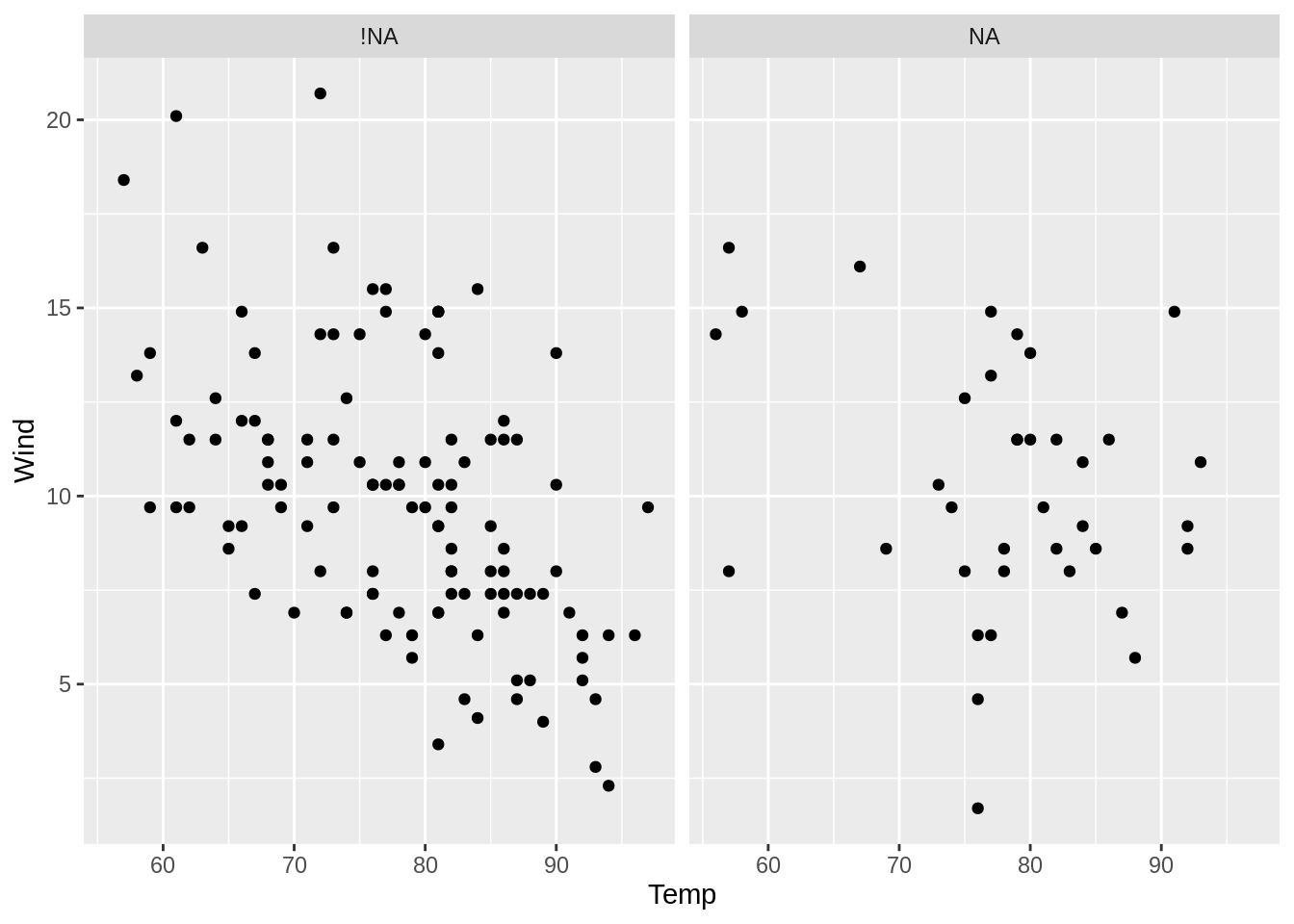Note there are fewer wind and temperature scores when ozone is missing, and that these tend to occur for temperatures over 70 and wind speeds over 5. Overall, the values of wind and temperature when ozone is missing seem similar to when ozone is present.

## 9.4 Visualizing missings using colour

Equivalently to the previous facetted plot, you can visualise the points according to whether they are missing.

``````airquality %>%
nabular() %>%
ggplot(aes(x = Temp,
y = Wind,
color = Ozone_NA)) +
geom_point()``````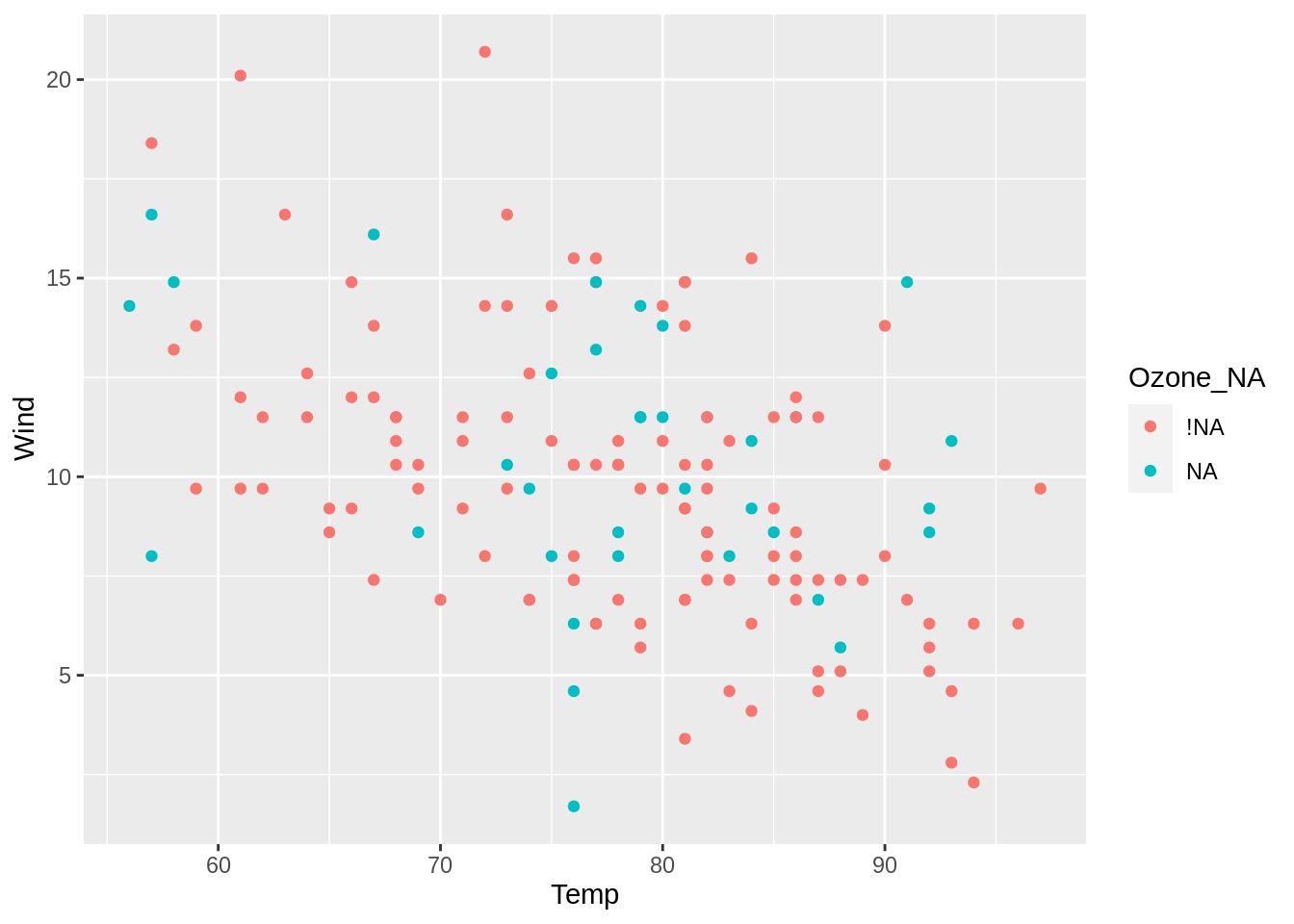This overlays the points rather than creating separate plots. This can sometimes help make comparisons easier, although this is not always the case. In the example above I cannot see any clear pattern in these points.

## 9.5 Adding layers of missingness

A useful advantage to using `facet` to split by missings is that this allows you to look at another condition of missingness. For example, create two plots by the missingness of solar radiation, and then colour the densities by missingness of ozone.

``````airquality %>%
nabular() %>%
ggplot(aes(x = Temp,
color = Ozone_NA)) +
geom_density()  +
facet_wrap(~Solar.R_NA)``````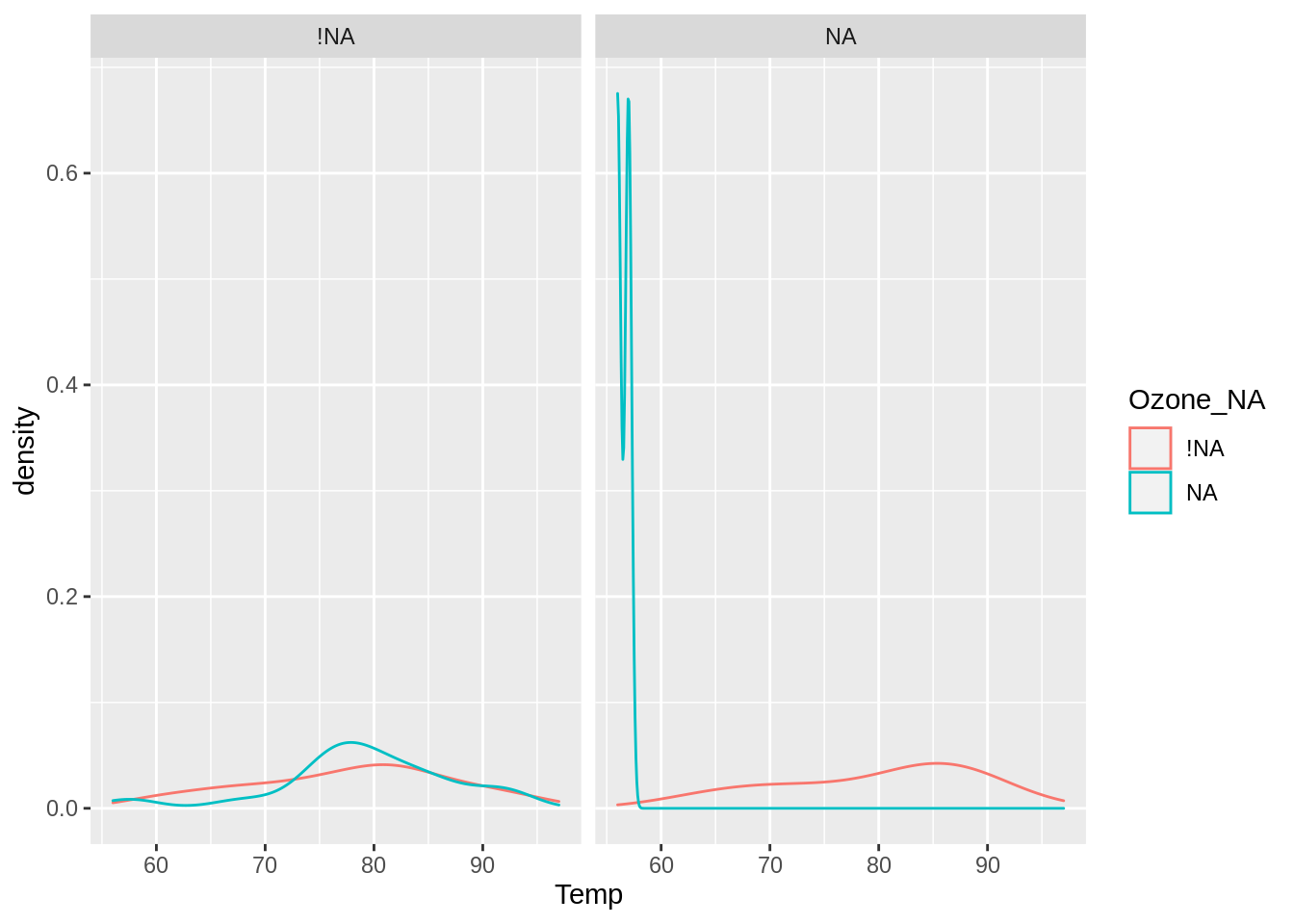This shows us that there isn’t much difference in temperature when solar radiation isn’t missing, but when solar radiation is missing, the temperatures are quite low!

Now that we’ve covered some methods for visually exploring missing data using `nabular` data and `ggplot2`, it’s time to practice using this on some other data.